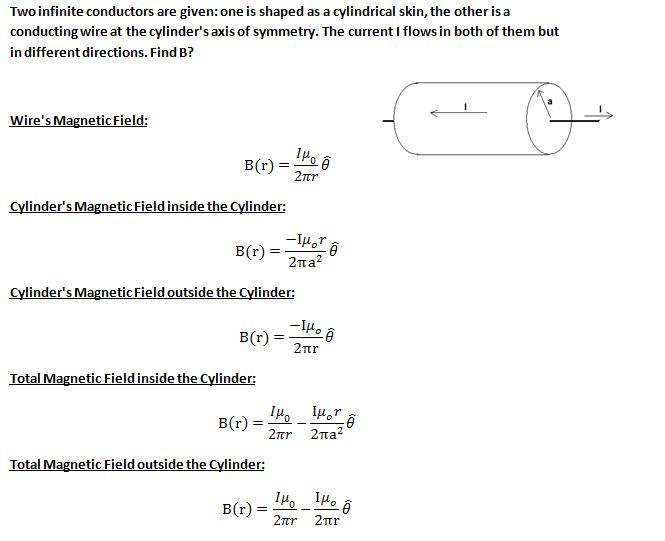# Amper's law superpositionthis is the wrong answer.... the question is where is my mistake...?

It's been awhile since I've done a problem like this, so please forgive me if I'm wrong.

The first thing you should do is draw two amperian loops. One inside the cylinder curling in the azimuthal direction with it's center being right where the wire is. The second one should be outside the cylinder with the same direction and same center as the first one.

Now the current in ampere's law is the current ENCLOSED by the amperian loops. So, for the one outside of everything the total current enclosed is zero (since the currents are in opposite directions). For the amperian loop inside the cylinder the current enclosed is I.

Therefore, the answer is, the field is zero outside the cylinder and inside the cylinder it is just the first field you gave (you called it the Wire's magnetic field). Is that the answer you were looking for?

Now, you might wonder why your answer is wrong. And I think they would be right if the conductors were not infinite, but they are and since they are we can use this amperian trick.